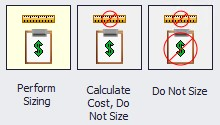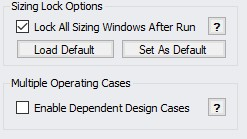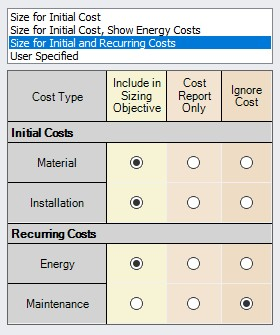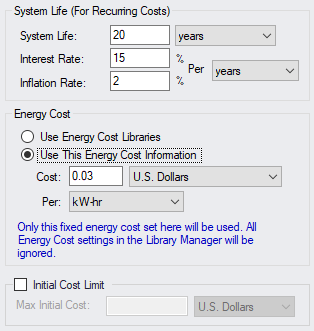#### Sizing Objective Panel

Sizing OptionsFigure 1: The three sizing ANS sizing methodologies are shown

• Perform Sizing - The best case selection of pipe sizes will be determined based on the various Sizing Window settings.

• Calculate Cost, Do Not Size - Pipe sizes will not change from their initial, user-defined size. The costs of the system as-defined will be calculated.

• Do Not Size - No sizing or cost calculations will be carried out. This is equivalent to temporarily disabling ANS.

Objective

The Objective is the goal of the automated sizing process. Any of the listed parameters can be minimized or maximized.

• Pipe Weight - Calculated using the pipe material density and dimensions from the internal pipe material library for the pipes being sized.

• Pipe and Fluid Weight - Calculated using the pipe material density and dimensions from the internal pipe material library, as well as the fluid density defined on the Fluid panel of Analysis Setup.

• Flow Volume - The total volume of fluid in the pipes calculated from the inner diameter and geometry defined in the Pipe Properties window.

• Pipe Inner Surface Area - The internal surface area of the pipes calculated using the inner diameter and geometry defined in the Pipe Properties window.

• Monetary Cost - The monetary value of the pipes/junctions calculated from the user provided cost libraries

Minimizing a physical parameter such as weight, volume, or surface area is often a good approximation of minimizing Initial Cost. These options are also useful in specific cases were monetary cost of the fluid system is not the primary concern.

Other OptionsFigure 2: The additional options in the Sizing window are shown

By default, all sizing panels are locked after a simulation is run. Modifying sizing input will require unlocking the panel.

It is possible to model multiple operating cases simultaneously by enabling Dependent Design Cases (Figure 2). The Dependent Design Cases panel is hidden unless it is enabled here.

Monetary Cost Options

There are several considerations to be made when considering the total Monetary Cost of a piping network. Is the Initial Cost of the Materials required the only concern? Do Installation Costs have to be accounted for? The true cost of a system could be considered its Life Cycle Cost - how much does it cost not only to build the system, but to run and maintain it? These are all valid approaches to the challenge of minimizing cost, and each requires different input.Figure 3: The various life cycle costs that can be considered are shown

• Size for Initial Cost - Material and Installation Costs will be used to size the system.

• Size for Initial Cost, Show Energy Costs - Material and Installation Costs will be used to size the system. Energy cost does not impact the sizing, but will be displayed. This may be helpful to determine if it is worthwhile to resize the system considering Recurring Costs.

• Size for Initial and Recurring Costs - Energy Cost is considered in the sizing, along with Material and Installation Cost.

• User Specified - Custom sizings are possible by selecting the appropriate radio buttons. For example, if Installation Costs are not readily available, these can be ignored by changing that row to Ignore Cost.

Note: The Sizing Level affects the available options. If the overall Sizing Level is "Do Not Size" then no sizing can take place and this will be reflected by forcing all cost rows to Ignore Cost.

• Warn When Fittings & Losses Costs are Missing - By default, ANS will warn the user whenever costs are missing for any Fitting & Loss defined within a sized pipe. For the most accurate solution, these costs should be included, however, they are often small compared to other costs and this option allows ignoring those warnings.

Recurring Cost Options

If any Recurring Costs is specified to be included in the Cost Report or Sizing, additional input is required (Figure 4).Figure 4: Additional required information when recurring costs are included

• System Life (For Recurring Costs) - The period for which the Recurring Costs are counted. Interest and Inflation Rates can optionally be specified.

• Energy Cost - Only enabled if Energy is included in the Cost Report or Sizing.

• Use Energy Cost Libraries - Use Cost Libraries for energy cost.

• Use This Energy Cost Information - Specify a monetary cost for energy directly. If this is specified, Energy Cost Libraries will not be utilized.

• Initial Cost Limit - Limits the total amount allowed for Initial Cost. A higher initial cost may result in a lower Life Cycle cost, but it is sometimes the case that there is an absolute limit on initial spending.

Related Blogs